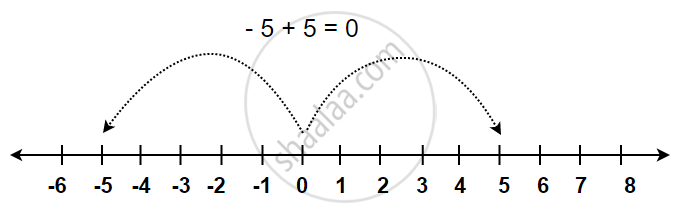#### definition

Additive Inverse: In mathematics, the additive inverse of a number a is the number that, when added to a yields zero. This number is also known as the opposite number. For example, - 5 + 5 = 0.

#### notesIn mathematics, the additive inverse of a number a is the number that, when added to a, yields zero. This number is also known as the opposite number.

For a real number, it reverses its sign: the opposite of a positive number is negative, and the opposite of a negative number is positive. Zero is the additive inverse of itself.

For example: - 5 + 5 = 0.

If you would like to contribute notes or other learning material, please submit them using the button below.